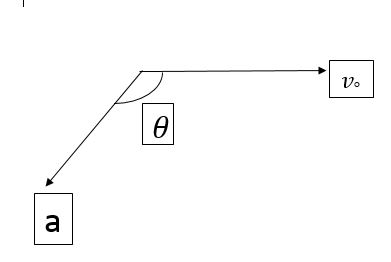# Projectile or Circular ?The figure shows the velocity and acceleration of a point like body at the intial moment of its motion. The acceleration vector of the body remains constant. The minimum radius of the curvature of trajectory of the body is :

Details and Assumptions

$v_{\circ} = 8 ms^{-1}$

$a = 2ms^{-1}$

$\theta = 150^{\circ}$

×